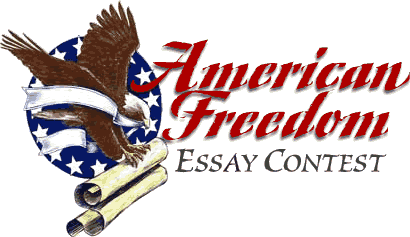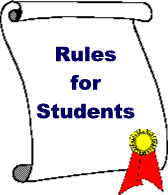This is an easy and effective way you can personally advance the cause of freedom.
Bring the American Freedom Essay Contest to your child's school --A wonderful learning  experience for students A no-cost enhancement  to the school year Real parent-teacher involvement
 "Let Freedom Ring"

THE AMERICAN FREEDOM ESSAY CONTEST
Executive Summary

This contest is designed to encourage school children and their teachers to think about the American concept of freedom, and its value to them. People who like the idea sponsor it, generally, for their own children's grade.

Basically, you offer a modest cash prize for the best essay, and two finalists each receive a smaller cash prize. The winners and the school are presented with parchment photo-reproductions of the Bill of Rights, and the winning student's teacher gets a framed certificate of achievement. Every student who enters the contest receives a frameable copy of the Bill of Rights. The size of the award is entirely up to you. A grand prize of \$100 and finalists at \$50 each has met with very enthusiastic responses from schools. Give more or less as you see fit.

A distinguished panel of reviewers, assembled locally, performs the judging after the school itself selects finalists. A straightforward set of rules describes the student entry requirements, the judging process, and the prize awards. Prizes are typically given at an awards assembly, and can be tied to a meaningful date in the country's history, or as an add-on to a prominent event already scheduled by the school. Local newspapers are encouraged to publish the winning essays, and copies can be posted on the world wide web.

Students compete within their grade (the whole grade participates), working from a set of questions. They cover one, any combination, or all of them, in essays ranging from 350 to 1,000 words:

What is freedom?

Is it important?

Where does freedom come from?

How free are we?

What role does government play in our freedom?

Are there threats to your individual freedom?

How can we preserve, protect and defend our freedom?

for your family, for America, and for the planet?

Schools benefit in many ways from participating in The American Freedom Essay Contest, and you are encouraged to consider this highly educational, strongly motivational, low-cost enhancement to the school year.

Say yes and help encourage the vitality of freedom for all people!

The American Freedom Essay Contest.
An idea with legs.Send us a Message

 WebMaster Contact:  webmaster@freedomessay.com

 x x x x x x x x x x x x x x x x x x x x x x x x x x x x x x x x x x x x x x x x x x x x x x x x x x x x x x x x x x x x x x x x x x x x x x x x x x x x x x x x x x x x x x x x x x x x x x x x x x x x x x x x x x x x x x x x x x x x x x x x x x x x x x x x x x x x x x x x x x x x x x x x x x x x x x x x x x x x x x x x x x x x x x x x x x x x x x x x x x x x x x x x x x x x x x x x x x x x x x x x x x x x x x x x x x x x x x x x x x x x x x x x x x x x x x x x x x x x x x x x x x x x x x x x x x x x x x x x x x x x x x x x x x x x x x x x x x x x x x x x x x x x x x x x x x x x x x x x x x x x x x x x x x